Home

# Quotients of A2 * T2

## Abstract

We study unitary quotients of the free product unitary pivotal category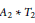${{A}_{2}}\,*\,{{T}_{2}}$ . We show that such quotients are parametrized by an integer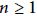$n\,\ge \,1$ and an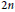$2n$ –th root of unity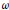$\omega$ . We show that for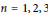$n\,=\,1,\,2,\,3$ , there is exactly one quotient and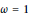$\omega \,=\,1$ . For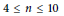$4\,\le \,n\,\le \,10$ , we show that there are no such quotients. Our methods also apply to quotients of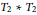${{T}_{2}}\,*\,{{T}_{2}}$ , where we have a similar result.

The essence of our method is a consistency check on jellyfish relations. While we only treat the specific cases of${{A}_{2}}\,*\,{{T}_{2}}$ and${{T}_{2}}\,*\,{{T}_{2}}$ , we anticipate that our technique can be extended to a general method for proving the nonexistence of planar algebras with a specified principal graph.

During the preparation of this manuscript, we learnt of Liu's independent result on composites of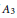${{A}_{3}}$ and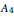${{A}_{4}}$ subfactor planar algebras (arxiv:1308.5691). In 1994, Bisch–Haagerup showed that the principal graph of a composite of${{A}_{3}}$ and${{A}_{4}}$ must fit into a certain family, and Liu has classified all such subfactor planar algebras. We explain the connection between the quotient categories and the corresponding composite subfactor planar algebras. As a corollary of Liu's result, there are no such quotient categories for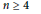$n\,\ge \,4$ .

This is an abridged version of arxiv:1308.5723.

## References

Hide All
MathJax
MathJax is a JavaScript display engine for mathematics. For more information see http://www.mathjax.org.

# Quotients of A2 * T2

## Metrics

### Full text viewsFull text views reflects the number of PDF downloads, PDFs sent to Google Drive, Dropbox and Kindle and HTML full text views.

Total number of HTML views: 0
Total number of PDF views: 0 *Loading metrics...

### Abstract viewsAbstract views reflect the number of visits to the article landing page.

Total abstract views: 0 *Loading metrics...

* Views captured on Cambridge Core between <date>. This data will be updated every 24 hours.

Usage data cannot currently be displayed.